Courses

# Motion Compulsory Test Class 9

## 20 Questions MCQ Test | Motion Compulsory Test Class 9

Description
This mock test of Motion Compulsory Test Class 9 for Class 9 helps you for every Class 9 entrance exam. This contains 20 Multiple Choice Questions for Class 9 Motion Compulsory Test Class 9 (mcq) to study with solutions a complete question bank. The solved questions answers in this Motion Compulsory Test Class 9 quiz give you a good mix of easy questions and tough questions. Class 9 students definitely take this Motion Compulsory Test Class 9 exercise for a better result in the exam. You can find other Motion Compulsory Test Class 9 extra questions, long questions & short questions for Class 9 on EduRev as well by searching above.
QUESTION: 1

Solution:
QUESTION: 2

Solution:
QUESTION: 3

### A body moving from rest with uniform acceleration travels a distance X in the first t seconds and travels a distance Y with same acceleration in the next 2t seconds, then

Solution:

For a body at rest, u = 0. Thus, we have

S = 1/2 at2

X = 1/2 at2

Y = 1/2 a (2t)2

= 1/2 a * 4t2

Y = 4x

QUESTION: 4

If a moving body comes to rest, then its acceleration is-

Solution:
QUESTION: 5

The equations of motion can be derived by using

Solution: The First Equation of Motion. Consider an object moving so that its velocity-time graph is a straight line. Such an object is undergoing constant acceleration, since the slope of the graph is constant. The first equation of motion gives the final velocity after a time t for these objects, given an initial velocity v0 .
QUESTION: 6

The third equation of motion is

Solution:
QUESTION: 7

A plank with a box on it at one end is gradually raised about the other end. As the angle of inclination with the horizontal reaches 30o, the box starts to slip and slides 4.0 m down the plank in 4.0 s. The coefficients of static and kinetic friction between the box and the plank will be respectively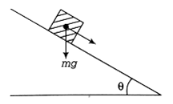Solution:

Given a plank with a box on it one end is gradually raised about the end having angle of inclination is 300, the box starts to slip and slides down  4 m the plank in 4 s as shown in a figure.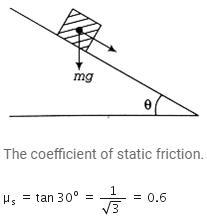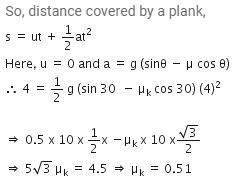Thus, coefficient of kinetic friction between the box and the plank is 0.51

QUESTION: 8

A cat runs around the boundary of a square field of side 6m in 10s. The displacement of the cat in 1 minute is

Solution: After running around the boundary of the square field, the cat reaches the same spot thus the displacement of the body is zero.
QUESTION: 9

The area under the speed-time graph gives the -----

Solution:
QUESTION: 10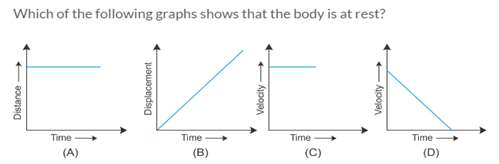Solution:
QUESTION: 11

An athlete uniformly increases his speed from rest at the rate of 1m/s2 .The time taken to complete 200m race is:

Solution:
QUESTION: 12

For a body starting from rest and moving with uniform acceleration, the ratio of the distances covered for 1s,2s,3s… is

Solution:
we need to use the formula, S = ut+(1/2)at^2, but initial velocity u = 0, hence S = (1/2)at^2

Distance covered in 1 sec S1 = (1/2)a ;
Distance covered in 2 sec S2 = (1/2)ax4  ;
Distance covered in 3 sec S3 = (1/2)ax9  ;

S1:S2:S3 = 1:4:9
QUESTION: 13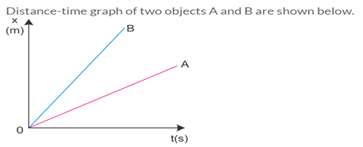Solution:
QUESTION: 14

Area under v-t graph represents a physical quantity which has the unit:

Solution:
QUESTION: 15

Name the instrument used to measure instantaneous speed of vehicle

Solution:
QUESTION: 16

If you move 5km north, 5km east and 5km south, then your displacement is

Solution:
QUESTION: 17

The ratio of CGS to MKS unit of acceleration is

Solution:

C.G.S unit of acceleration = 1 cm/s2

M.K.S. unit of acceleration = 1m/s2

C.G.S unit of acceleration/ M,K.S unit of acceleration =

1cm/s2 / 1m/s2

= 1cm / 100 cm

= 1/100

=1:100

QUESTION: 18

If a train is travelling due north and its braked are applied, it will have?

Solution:
QUESTION: 19

A car increases its speed from 20km/h to 40km/h in 10 seconds. Its acceleration is

Solution:
QUESTION: 20

If the speed-time graph is a straight line parallel to the time axis, then the body is

Solution: Speed-time graph is a straight line parallel to time axis, it means that the speed of the object is not changing with time i.e., the object is performing uniform motion.